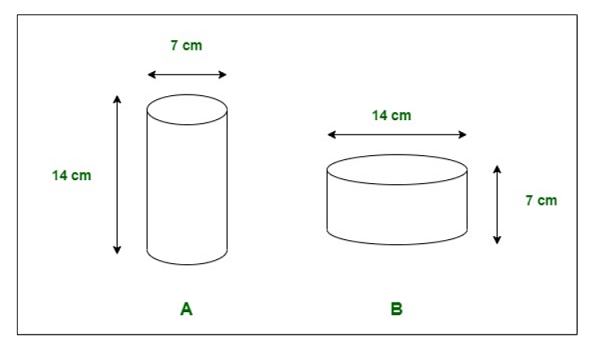Class 8 NCERT Solutions – Chapter 11 Mensuration – Exercise 11.4

• Difficulty Level : Hard
• Last Updated : 20 Nov, 2020

Question 1. Given a cylindrical tank, in which situation will you find the surface area and in which situation volume

(a) To find how much it can hold.
(b) Number of cement bags required to plaster it.
(c) To find the number of smaller tanks that can be filled with water from it.

Solution:

a) In this condition, we will find the volume of a cylindrical tank.

b) In this condition, we will find the surface area of a cylindrical tank.

c) In this condition, we will find the volume of a cylindrical tank.

Question 2. The diameter of cylinder A is 7 cm, and the height is 14 cm. The diameter of cylinder B is 14 cm and the height is 7 cm. Without doing any calculations can you suggest whose volume is greater? Verify it by finding the volume of both the cylinders. Check whether the cylinder with greater volume also has a greater surface area?Solution:

Cylinder A:

Radius of cylinder A = 7/2 cm

Height of cylinder A = 14 cm

Volume of cylinder A = pi.r2h = 22/7 x 7/2 x 7/2 x 14 = 539 cm3

Total Surface area of cylinder A = 2.pi.r(h + r) = 2 x 22/7 x 7/2(14 + 7/2) = 385 cm2

Cylinder B:

Radius of cylinder B = 14/2 or 7 cm

Height of cylinder B = 7 cm

Volume of cylinder B = pi.r2h = 22/7 x 7 x 7 x 7 = 1078 cm3

Total Surface area of cylinder B = 2.pi.r(h + r) = 2 x 22/7 x 7(7 + 7) = 616 cm2

We can clearly see that the Volume of cylinder B is twice that of cylinder A. Hence, verified that the Volume of cylinder B is greater than the volume of cylinder A. Also, Total surface area of cylinder B is greater.

Question 3. Find the height of a cuboid whose base area is 180 cm2 and volume is 900 cm3?

Solution:

Area of Cuboid = length x breadth = 180 cm2

Volume of Cuboid = length x breadth x height = 900 cm3

So, Area x height = Volume

180 cm2 x height = 900 cm3

Hence, height = 5 cm

Hence, height of given cuboid is 5 cm.

Question 4. A cuboid is of dimensions 60 cm × 54 cm × 30 cm. How many small cubes with side 6 cm can be placed in the given cuboid?

Solution:

Volume of Cuboid = length x breadth x height = 60 cm x 54 cm x 30 cm = 97200 cm3

Volume of Cube = (side)3 = (6cm)3 = 216 cm3

Number of small cubes that can be placed inside given cuboid = Volume of given Cuboid/ Volume of one Cube

= 97200/216

= 450

Hence, 450 small cubes can be placed inside given cuboid.

Question 5. Find the height of the cylinder whose volume is 1.54 m3 and diameter of the base is 140 cm?

Solution:

Volume of cylinder = 1.54 m3

Diameter of base of cylinder = 140 cm = 1.40 m, Radius = 7.20 m

As we know, Volume of cylinder = pi.r2h

So, 1.54 = 22/7 x 7.2 x 7.2 x height

On doing calculation, we get Height of cylinder = 1 m

Hence, height of given cylinder is 1 m.

Question 6. A milk tank is in the form of cylinder whose radius is 1.5 m and length is 7 m. Find the quantity of milk in litres that can be stored in the tank?

Solution:

The radius of the cylindrical tank = 1.5 m

Length/Height of cylindrical = 7 m

The quantity of milk in liters that can be stored in the tank = Volume of the cylindrical tank

So, Volume of cylinder = pi.r2h = 22/7 x 1.5 x 1.5 x 7 = 49.50 m3

Volume = 49.50 m3

As we know, 1 m3 = 1000 liters

So, 49.50 m3 = 49500 L

Hence, the quantity of milk in liters that can be stored in the tank is 49500 L

Question 7. If each edge of a cube is doubled,

(i) how many times will it be surface area increase?
(ii) how many times will its volume increase?

Solution:

Let us assume that the original edge of the cube is a cm. If the edge of the cube is doubled, then the new edge will be 2a cm.

i) Original surface area of cube = (edge)2 = (a)2 = a2 cm2

New surface area of cube = (edge)2 = (2a)2 = 4a2 cm2

Ratio to find which cube’s surface area is greater = Original surface area : New surface area = a2 : 4a2 = 1 : 4

Hence, surface area of cube is increased by 4 times.

ii) Original volume of cube = (edge)3 = (a)3 = a3 cm3

New volume of cube = (edge)3 = (2a)3 = 8a3 cm3

Ratio to find which cube’s volume is greater = Original volume : New volume = a3 : 8a3 = 1 : 8

Hence, volume of cube is increased by 8 times.

Question 8. Water is pouring into a cuboidal reservoir at the rate of 60 litres per minute. If the volume of the reservoir is 108 m3, find the number of hours it will take to fill the reservoir.

Solution:

Given the volume of the reservoir is 108 m3 Or 108000 L [1 m3 = 1000 L]. The volume of water pouring into a cuboidal reservoir = 60 L / minutes.

So, the time is taken to fill the reservoir = Volume of cuboidal reservoir / Volume of water pouring into the reservoir per minute

= 108000/60

= 1800 minutes

= 1800/60

= 30 hours

Hence, it will require 30 hours to fill the reservoir completely.

My Personal Notes arrow_drop_up